Free Printable Worksheets for Fourth grade

## Algebra: 1 Step Addition & Subtraction Equations (Set 4)Free algebra worksheet – 1 step addition and subtraction equations. – 15 problems per page – solutions included...

## Algebra: 1 Step Addition & Subtraction Equations (Set 3)Intro algebra worksheet: Find the value of x for the problems listed. – 1 step addition and subtraction equations. – with solutions...

## Algebra: 1 Step Addition & Subtraction Equations (Set 2)Algebra introduction worksheet. 1 step addition and subtraction equations. – 15 problems with solutions...

## Algebra: 1 Step Addition & Subtraction Equations (Set 1)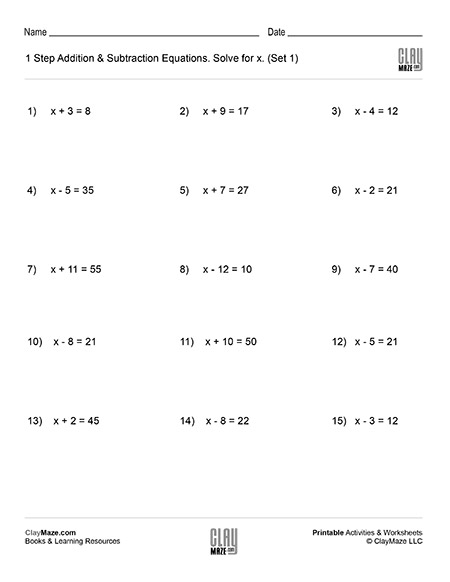This worksheet contains problems that act as an introduction to algebra. The problems are 1 step addition and subtraction equations that ask the student to solve for x. – solutions included...

## Basic Statistics: Probability I (Set 4)Worksheet on basic probability – The student is asked several questions about the number of shapes in the box and to find the probabilities of selecting different shapes at random....

## Basic Statistics: Probability I (Set 3)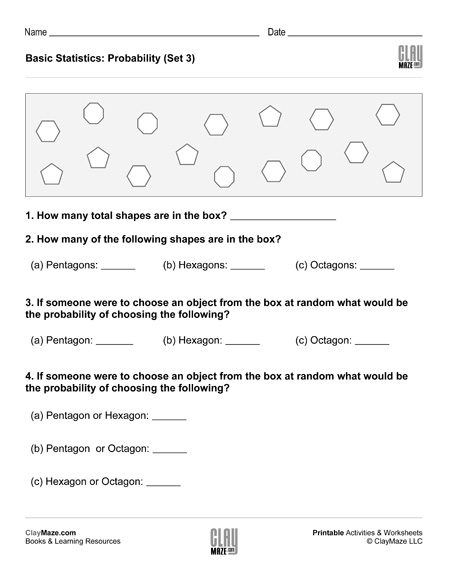This is a basic probability worksheet. The questions are based on the number of shapes in the box and the student is asked to find the probabilities of randomly choosing the different shapes....

## Basic Statistics: Probability I (Set 2)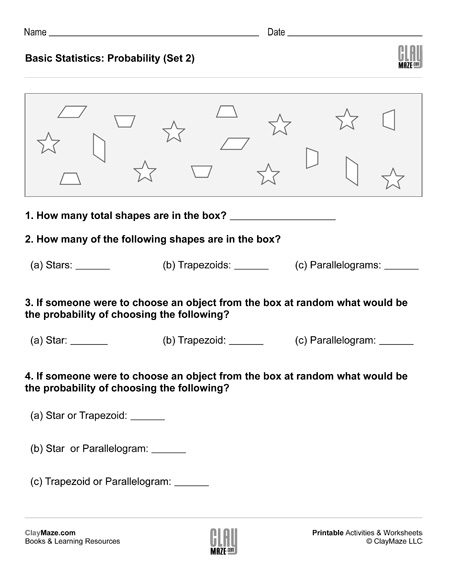This free worksheet covers basic probability. The student is asked several questions about the number of shapes in the box. The student can then use those values to find the probabilities of...

## Basic Statistics: Probability I (Set 1)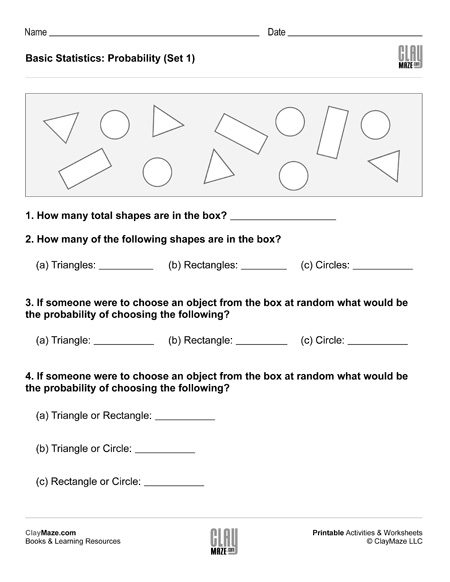This statistics worksheet is on basic probability. There are a number of different shapes in the box and the student is asked to find the probabilities of selecting the different shapes at random....

## Statistics: Minimum, Maximum, Mean, Median, Mode, Range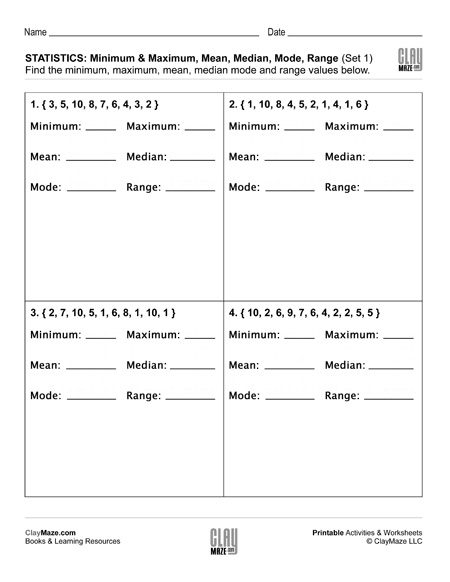Each of the statistics worksheets in this set contains 4 sets of data. The student is asked to find the minimum, maximum, mean, median, mode and range for each data set. These are recommended...

## Reading Comprehension Word Problem: Lab Experiment 3This free children’s worksheet is the 3rd part of a 3 part series which combines scientific experiment with word problems.  Exercises like these are a great bridge between early learning...

## Geometry: Find the Missing Angle in the Triangle (Set 4)In this worksheet the student is asked to find the missing angle in the triangles shown. This sheet is for students that are familiar with right, scalene and isosceles triangles....

## Geometry: Find the Missing Angle in the Triangle (Set 3)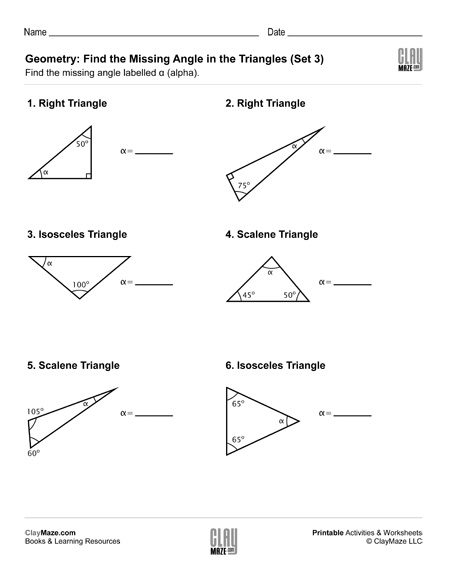Geometry worksheet on triangles and angles. The student is asked to find the unknown angle using their knowledge of triangles....

## Geometry: Find the Missing Angle in the Triangle (Set 2)Download this worksheet on finding the missing angle in a triangle. This worksheet assume the student is familiar with right, isosceles and scalene triangles and knows that the angles in a...

## Geometry: Find the Missing Angle in the Triangle (Set 1)This worksheet is for finding a missing angle in a triangle and is based on the student knowing that there are 180 degrees in a triangle. It also assumes the student knows the different types of...

## Order of Operations I (Set 4)Print this worksheet for practice using the correct order of operations. The problems use a combination of addition, subtraction, multiplication and division....